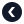Python专题Python 语句

2021-07-02

语句

age = 20
if age >= 18:
print("已成年")

打印输出

print("我正在学习Python")

续行符

total = 1.2 + 3.5 + 4.9 + \
2.4 + 6.6 + 3.2 + \
3.3 + 4.5 + 6.5

total = 1.2 + 3.5 + 4.9 + 2.4 + 6.6 + 3.2 + 3.3 + 4.5 + 6.5

隐式续航

total = (1.2 + 3.5 + 4.9 +
2.4 + 6.6 + 3.2 +
3.3 + 4.5 + 6.5)

语句的结尾

Python和JavaScript语言一样，对于一句语句的结尾可以加分号作为语句的结束标记，也可以省略语句结尾的分号。

小练习

1. 尝试去掉上面沙盒中的语句之间的;，看看运行是否会出错？
2. 尝试把原来有;的地方改成换行并移除;，看看运行结果是否依然正常？